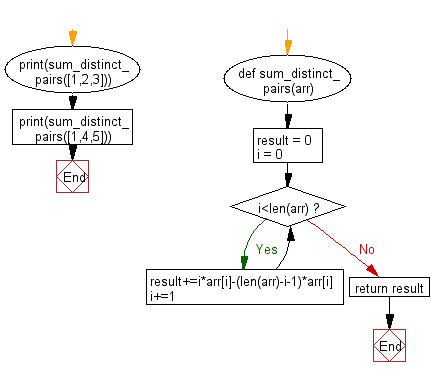﻿ Python: Compute the summation of the absolute difference of all distinct pairs in a given array - w3resource# Python: Compute the summation of the absolute difference of all distinct pairs in a given array

## Python Basic - 1: Exercise-26 with Solution

Write a Python program to compute the summation of the absolute difference of all distinct pairs in a given array (non-decreasing order).

Sample array: [1, 2, 3]
Then all the distinct pairs will be:
1 2
1 3
2 3

Sample Solution:

Python Code:

``````def sum_distinct_pairs(arr):
result = 0
i = 0
while i<len(arr):
result+=i*arr[i]-(len(arr)-i-1)*arr[i]
i+=1
return result
print(sum_distinct_pairs([1,2,3]))
print(sum_distinct_pairs([1,4,5]))
``````

Sample Output:

```4
8
```

Pictorial Presentation:Flowchart:## Visualize Python code execution:

The following tool visualize what the computer is doing step-by-step as it executes the said program:

Python Code Editor:

Have another way to solve this solution? Contribute your code (and comments) through Disqus.

What is the difficulty level of this exercise?

Test your Programming skills with w3resource's quiz.

﻿

## Python: Tips of the Day

What is the difference between Python's list methods append and extend?

append: Appends object at the end.

```x = [1, 2, 3]
x.append([4, 5])
print (x)
```

Output:

```[1, 2, 3, [4, 5]]
```

extend: Extends list by appending elements from the iterable.

```x = [1, 2, 3]
x.extend([4, 5])
print (x)
```

Output:

```[1, 2, 3, 4, 5]
```

Ref: https://bit.ly/2AZ6ZFq